SEARCH HOMEMath Central Quandaries & QueriesQuestion from sasha, a student: Hi, I need help with the following question. I've to find the power series solution of the differential equation: y' = xy. I don't know how to find the recursive equation. Can you please help me. ThanksSasha,

Suppose the solution is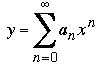then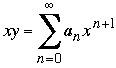and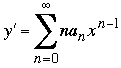. Hence if y' = xy then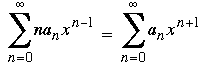For the series on the right side change the index to k and rewrite it as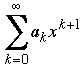The left side can be written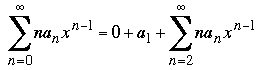Let k = n - 2 and this becomes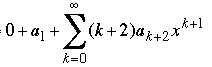Hence y' = xy can be written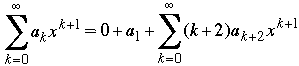Now you can see the coefficients that must be equal

ak = (k + 2)ak+2 for k = 0, 1, 2 ···

I hope this helps,
HarleyMath Central is supported by the University of Regina and The Pacific Institute for the Mathematical Sciences.